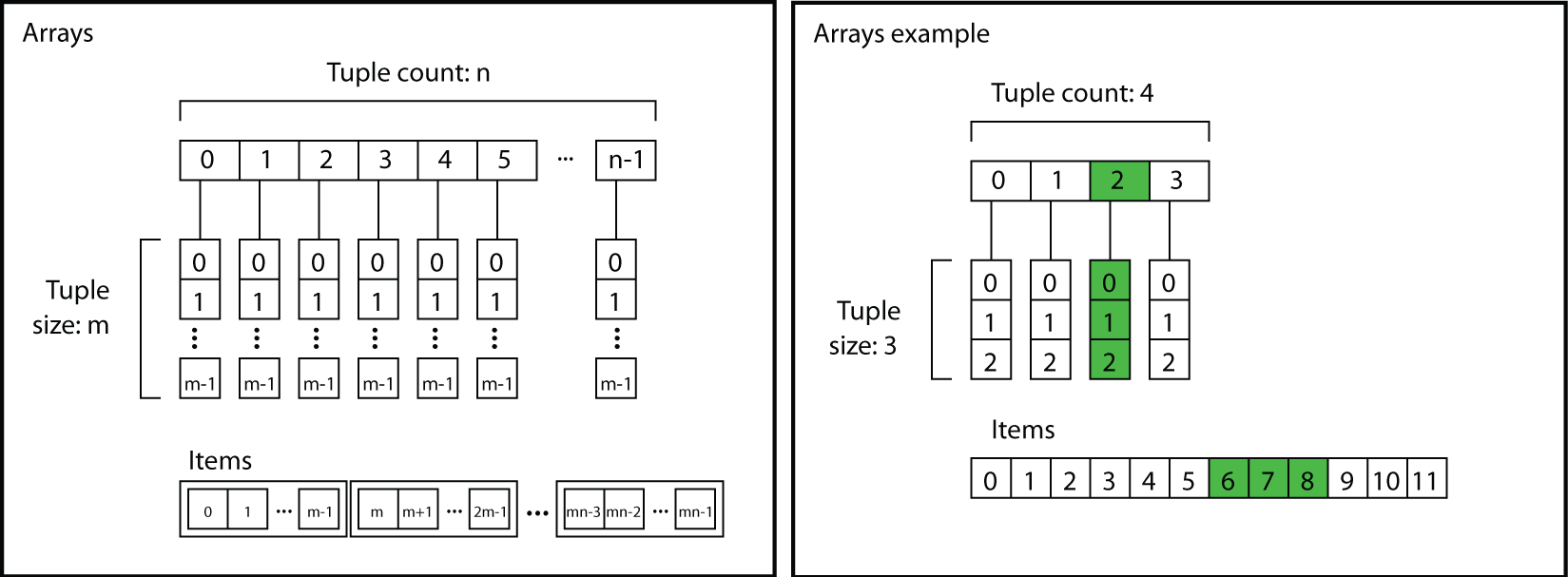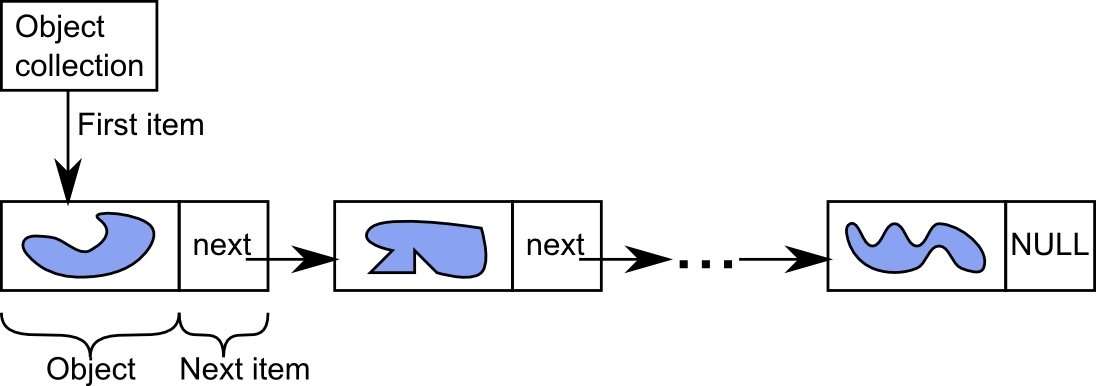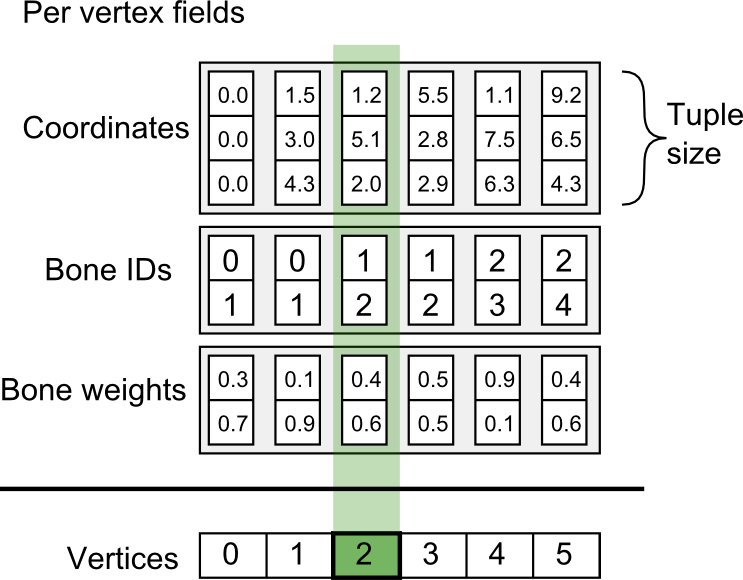# # Data classes

## # Array types

The Simplygon API provides several array container types. These array types are named IArray, where denotes the type of elements stored in the array. The most commonly used array types are IRealArray and IRidArray that are used for most of the communication between the user and the API. The arrays store the elements as tuples of user-specified size. This is used extensively in Simplygon to store e.g. 3D vectors in an array, where each vector is stored in a tuple, and the number of tuples in the array equals the number of vectors. The offset in the array to a certain item in a tuple can be computed as:

``````offset = tupleindex * tuplesize + itemindex
``````

The array interface also provides an interface for working directly with the tuples.### # Data types

There are data classes for each array type, e.g. there is an spRealData for the spRealArray and so on. The data objects exist to ensure proper range checking is used when accessing the Simplygon array data. Having the data object allows us to fetch or set data at a certain location in the array. For instance, calling the spRealArray::GetData(spRealData) or the spRealArray::GetTuple(int, spRealData) function will give the data object the pointer to the array's data. Once the Data object has been initialized with the array pointer, calling the data object's GetItemCount() will return the array's total data count or its tuple size, depending on which one of GetData() or GetTuple() was called.

### # Example - Array creation

The following code shows how to create an array and fill it with some vector data.

### # Example - Array iteration

The following code listing shows how to iterate over all the tuple elements in an array and printing each tuple on its own line.

### # Example - Using a Data object

The following code shows how to use a Data object to fetch the raw data from an array. This is automatically modified into a standard array in Python and C#.

## # Object collection types

The Simplygon API provides a special container type for storing Simplygon objects. These container types are referred to as object collections.

The collection types use the same naming scheme as the array types. The most generic object collection type is named IObjectCollection and is capable of storing anything that derives from the Simplygon IObject class and implements the required interface. The object containers use the rhandle type for referencing items in them so iteration over the elements is done using the GetFirstItem and GetNextItem methods. For simplicity, there are some collections derived from IObjectCollection, such as IGeometryDataCollection, that keeps object of type IGeometryData. These derived classes has methods for directly retrieving the object type, such as GetGeometryData instead of using GetItemsObject, and then casting the returned pointer.### # Example - Working with object collections

This example shows how to work with object collections.

## # Field data objects

Field data objects are properties stored in arrays either per triangle, vertex or triangle corner. Examples of array data stored per vertex is: coordinates, texture coordinates (continuous between triangles), bone IDs and bone weights. Vertex and material IDs are examples of information stored per triangle. Corners store normals and texture coordinates when they are discontinuous between triangles.

The user can create data arrays and decide if they are per vertex, triangle or corner. The data is stored in arrays with the tuple count being the size of the field it's assigned to. For instance, the vertex coordinates data array has a tuple count of the number of vertices and tuple size three (because of 3d Cartesian coordinates).